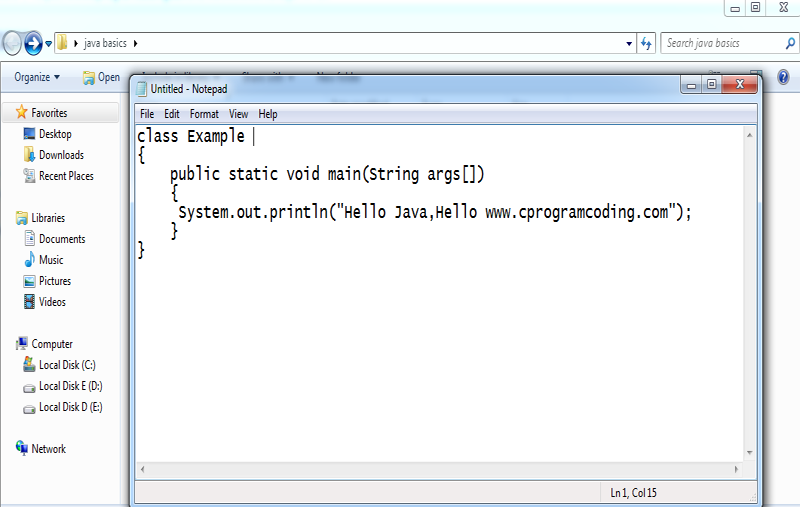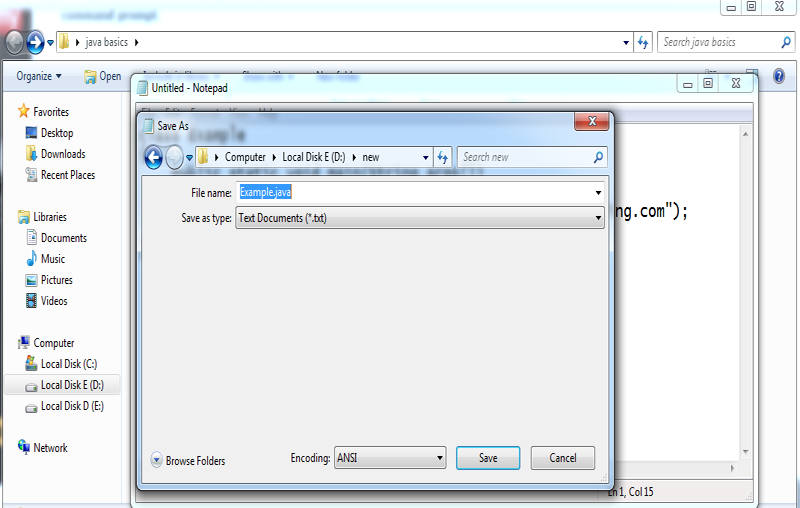# Java Tutorial

## Simple Program of Java

Java programming: Java program code consists of instructions which will be executed on your computer system to perform a task as an example say arrange given integers in ascending order. This page contains sample programs for beginners to understand how to use Java programming to write simple Java programs. These programs demonstrate how to get input from a user, working with loops, strings, and arrays. Programs are provided with the output (image file), and you can also download class file and execute it directly without compiling the source file.

##### Requirement for Hello Java Example

For executing any java program, you need to

1. install the JDK if you don't have installed it, download the JDK and install it.

2. set path of the jdk/bin directory.
3. create the java program
4. compile and run the java program
##### Creating hello java example
```
class Simple
{
public static void main(String args[])
{
System.out.println("Hello Java");
}
}
```

save this file as Simple.java

To compile: javac Simple.java

To execute: java Simple

##### Output:
```

Output:Hello Java

```
##### Parameters used in first java program

Let's see what is the meaning of class, public, static, void, main, String[], System.out.println().

• class keyword is used to declare a class in java.

• public keyword is an access modifier which represents visibility, it means it is visible to all.

• static is a keyword, if we declare any method as static, it is known as static method. The core advantage of static method is that there is no need to create object to invoke the static method. The main method is executed by the JVM, so it doesn't require to create object to invoke the main method. So it saves memory.

• void is the return type of the method, it means it doesn't return any value.

• main represents the starting point of the program.

• String[] args is used for command line argument. We will learn it later.

• System.out.println() is used print statement. We will learn about the internal working of System.out.println statement later.

To write the simple program, open notepad by start menu -> All Programs -> Accessories -> notepad and write simple program as displayed below:As displayed in the above diagram, write the simple program of java in notepad and saved it as Simple.java. To compile and run this program, you need to open command prompt by start menu -> All Programs -> Accessories -> command prompt.To compile and run the above program, go to your current directory first; my current directory is c:\new . Write here:

To compile: javac Simple.java

To execute: java Simple

##### How many ways can we write a java program

There are many ways to write a java program. The modifications that can be done in a java program are given below:

##### 1) By changing sequence of the modifiers, method prototype is not changed.

The simple code of main method.

```
static public void main(String args[])
```

##### 2) subscript notation in java array can be used after type, before variable or after variable.

The different codes to write the main method.

```
public static void main(String[] args)
public static void main(String []args)
public static void main(String args[])
```

##### 3) You can provide var-args support to main method by passing 3 ellipses (dots)

The simple code of using var-args in main method. We will learn about var-args later in Java New Features chapter.

```
public static void main(String... args)
```

##### 4) Having semicolon at the end of class in java is optional.

The simple code.

```
class A
{
static public void main(String... args){
System.out.println("hello java4");
}
};
```

##### Valid java main method signature
```
public static void main(String[] args)
public static void main(String []args)
public static void main(String args[])
public static void main(String... args)
static public void main(String[] args)
public static final void main(String[] args)
final public static void main(String[] args)
final strictfp public static void main(String[] args)
```

##### Invalid java main method signature
```
public void main(String[] args)
static void main(String[] args)
public void static main(String[] args)
abstract public static void main(String[] args)
```

##### Resolving an error "javac is not recognized as an internal or external command" ?

If there occurs a problem like displayed in the below figure, you need to set path. Since DOS doesn't know javac or java, we need to set path. Path is not required in such a case if you save your program inside the jdk/bin folder. But its good approach to set path. Click here for How to set path in java.

-->>> how to resolve the problem of simple program in java ------->>